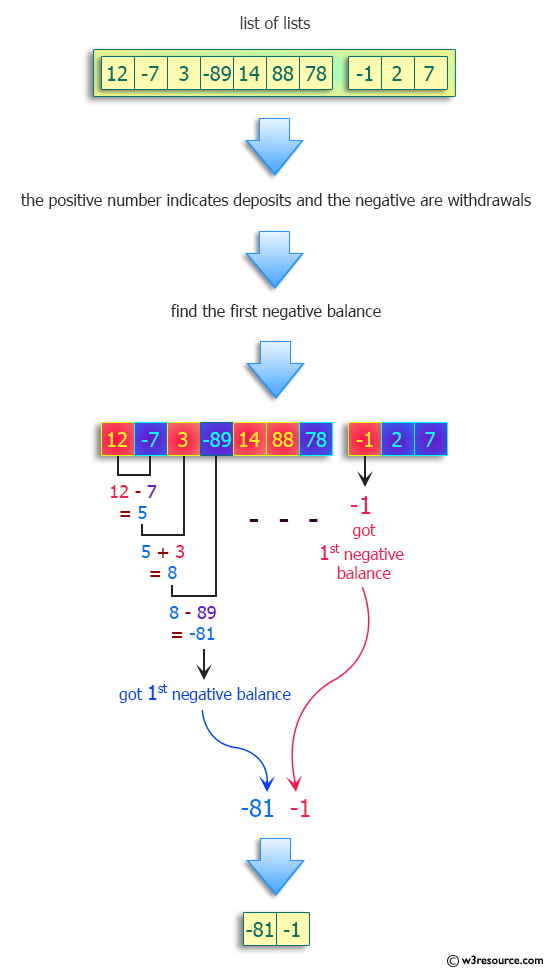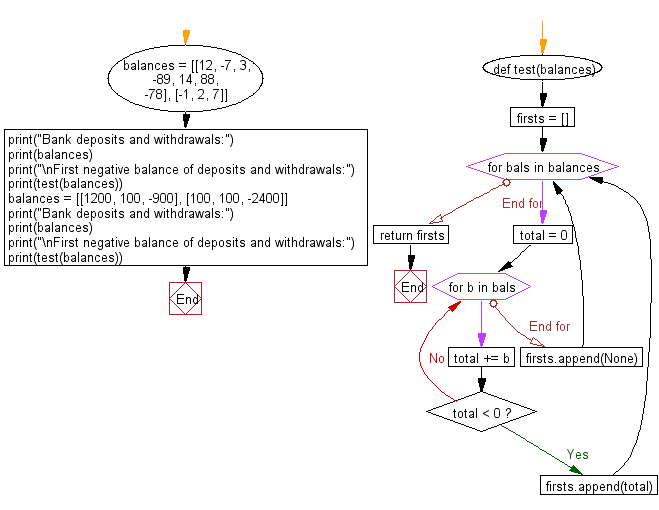﻿ Python: Find the first negative balance - w3resource# Python: Find the first negative balance

## Python Programming Puzzles: Exercise-70 with Solution

Write a Python program to find the first negative balance from a given a list of numbers which represent bank deposits and withdrawals.

```Input:
[[12, -7, 3, -89, 14, 88, -78], [-1, 2, 7]]

Output:
[-81, -1]
Input:
[[1200, 100, -900], [100, 100, -2400]]

Output:
[None, -2200]
```

Pictorial Presentation:Sample Solution:

Python Code:

``````#License: https://bit.ly/3oLErEI
def test(balances):
firsts = []
for bals in balances:
total = 0
for b in bals:
total += b
if total < 0:
firsts.append(total)
break
else:
firsts.append(None)
return firsts
balances = [[12, -7, 3, -89, 14, 88, -78], [-1, 2, 7]]
print("Bank deposits and withdrawals:")
print(balances)
print("\nFirst negative balance of deposits and withdrawals:")
print(test(balances))
balances = [[1200, 100, -900], [100, 100, -2400]]
print("Bank deposits and withdrawals:")
print(balances)
print("\nFirst negative balance of deposits and withdrawals:")
print(test(balances))
``````

Sample Output:

```Bank deposits and withdrawals:
[[12, -7, 3, -89, 14, 88, -78], [-1, 2, 7]]

First negative balance of deposits and withdrawals:
[-81, -1]
Bank deposits and withdrawals:
[[1200, 100, -900], [100, 100, -2400]]

First negative balance of deposits and withdrawals:
[None, -2200]
```

Flowchart:## Visualize Python code execution:

The following tool visualize what the computer is doing step-by-step as it executes the said program:

Python Code Editor :

Have another way to solve this solution? Contribute your code (and comments) through Disqus.

What is the difficulty level of this exercise?

Test your Programming skills with w3resource's quiz.

﻿

## Python: Tips of the Day

Clamps num within the inclusive range specified by the boundary values x and y:

Example:

```def tips_clamp_num(num,x,y):
return max(min(num, max(x, y)), min(x, y))
print(tips_clamp_num(2, 4, 6))
print(tips_clamp_num(1, -1, -6))
```

Output:

```4
-1
```